# VSEPR GEOMETRIES VSEPR Geometries 2 lone pairs Steric No. Basic Geometry O lone pair 1 lone...

VSEPR GEOMETRIES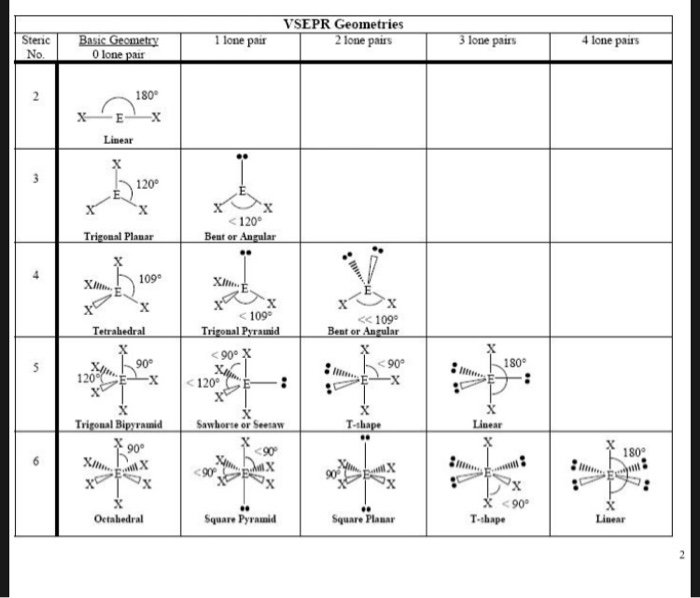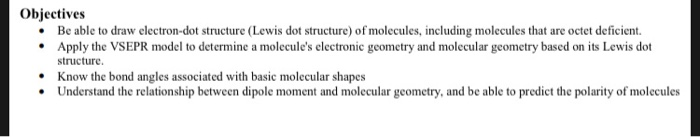VSEPR Geometries 2 lone pairs Steric No. Basic Geometry O lone pair 1 lone pair 4 lone pair's 3 lone pairs 180° Linear <120 Bent or Angular Planar X Tetrahedral << 109° Beat or Angular <109° Trigonal Pyramid < 90° X XI <120° CE- 120°CX Trigonal Bipyramid Sawbore or Seesaw 190° Wa 2 T 90° * T-shape tabedral Square Pyramid Square Planar
Objectives Be able to draw electron-dot structure (Lewis dot structure) of molecules, including molecules that are octet deficient. Apply the VSEPR model to determine a molecule's electronic geometry and molecular geometry based on its Lewis dot structure. Know the bond angles associated with basic molecular shapes Understand the relationship between dipole moment and molecular geometry, and be able to predict the polarity of molecules

SIMPLEST TRICK TO DETERMINE THE GEOMETRY AND SHAPE TO FIND GEOMETRY AND SHAPE

1. Calculate the valence electron

2. if valence electron count is less than or equal to 8 divides it by 2

if valence electron count is less than or equal to 56 divides it by 8

if valence electron count is greater than or equal to 56 divides it by 18

3. Predict the geometry & shape by using the table given below as provided in the question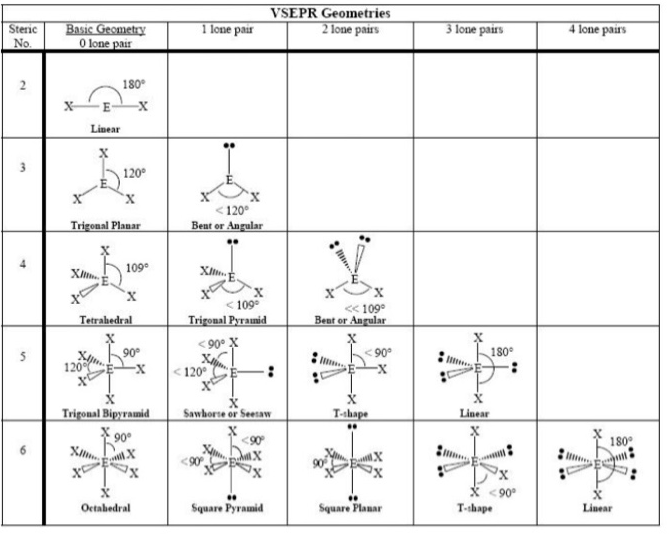Order of repulsion : bond pair-bond pair < bond pair-lone pair < lone pair-lone pair

Based on these factors bond angles distorts in a given structure

#### Earn Coin

Coins can be redeemed for fabulous gifts.

Similar Homework Help Questions
• ### Predict the electron pair geometry and the molecular structure for the polyatomic ion: BrCla octahedral -...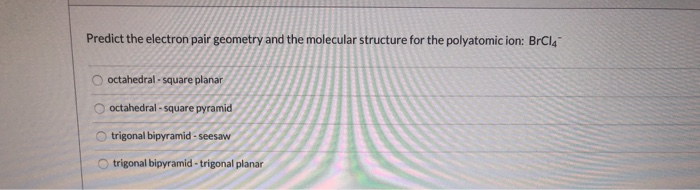Predict the electron pair geometry and the molecular structure for the polyatomic ion: BrCla octahedral - square planar octahedral - square pyramid trigonal bipyramid - seesaw trigonal bipyramid - trigonal planar

• ### Draw the Lewis structure of CIBr, showing all lone pairs. Identify the molecular geometry of CIBry...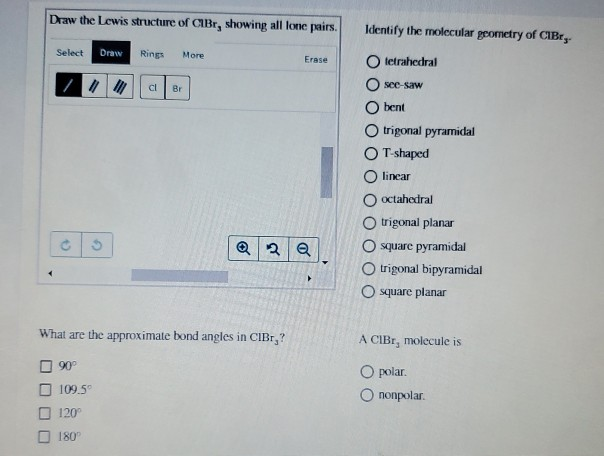Draw the Lewis structure of CIBr, showing all lone pairs. Identify the molecular geometry of CIBry Select Draw Rings More Erase tetrahedral see-saw bent trigonal pyramidal T-shaped O linear octahedral trigonal planar O square pyramidal trigonal bipyramidal square planar What are the approximate bond angles in CIBr,? A CIBr, molecule is O polar. Ononpolar. 90° 109.5 120 180

• ### Draw the Lewis structure of XeCl. Include all lone pairs. Identify the molecular geometry of Xeci,...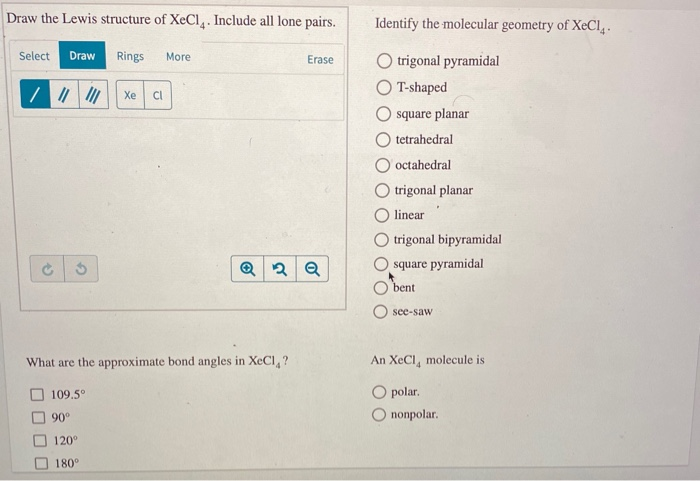Draw the Lewis structure of XeCl. Include all lone pairs. Identify the molecular geometry of Xeci, Select Draw Rings More Erase trigonal pyramidal T-shaped square planar O tetrahedral O octahedral trigonal planar linear trigonal bipyramidal square pyramidal bent O see-saw What are the approximate bond angles in XeCl,? An XeCl, molecule is O polar O nonpolar. 109.5° 90° 120° 180°

• ### 2. Why do actual bond angles differ from the ideal bond angles? How does a lone...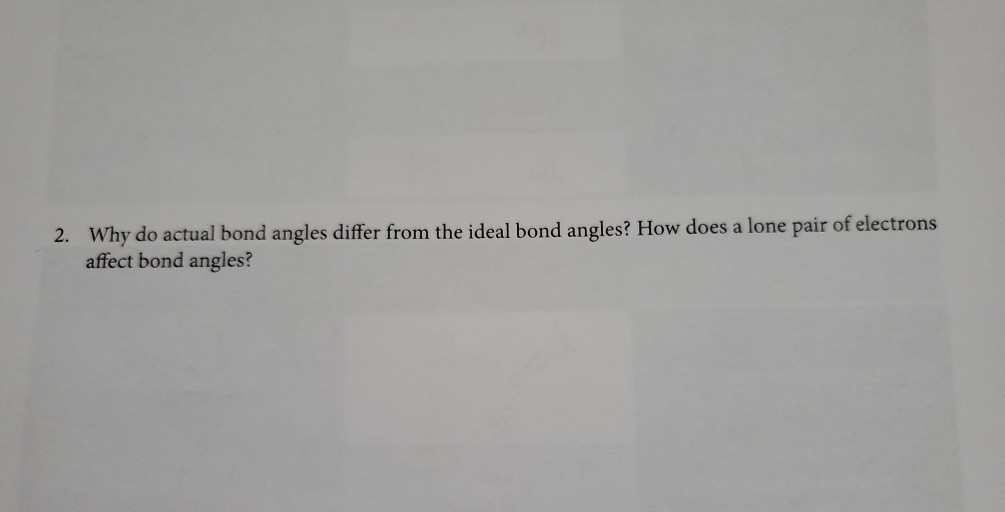2. Why do actual bond angles differ from the ideal bond angles? How does a lone pair of electrons affect bond angles? Lewis Dot structure Electronic geometry Molecular shape Ideal Bond angles Hybridization of central atom Does it contain polar bonds? Is the molecule polar? NH NH NH; tetrahedral Trigonal pyramidal 109 Spyes yes PES trigonal bipyramidal trigonal bipyramid Sp'd yes no and 90 Square Bris 90 and Octahedral Sn y es pyramidal yes 180 HCN HỮc : | linear...

• ### 34. The hybridization on the oxygen atom in H3O* is best described as a. sp b....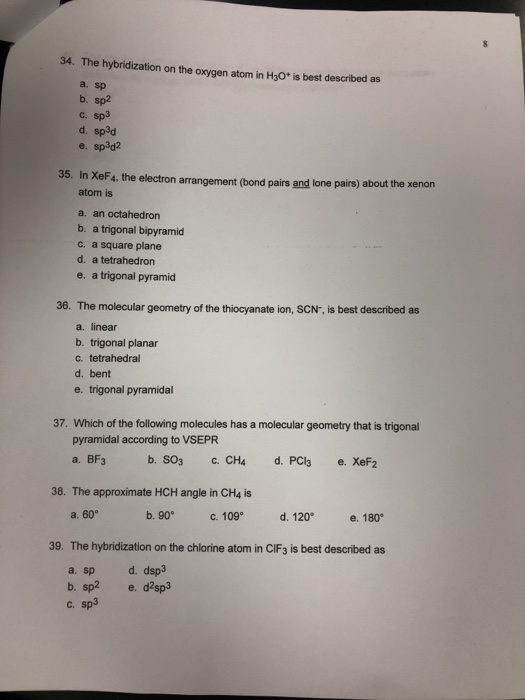34. The hybridization on the oxygen atom in H3O* is best described as a. sp b. sp2 c. sp3 d. sp3d e. sp3d2 35. In XeF4, the electron arrangement (bond pairs and lone pairs) about the xenon atom is a. an octahedron b. a trigonal bipyramid c. a square plane d. a tetrahedron e. a trigonal pyramid 36. The molecular geometry of the thiocyanate ion, SCN-, is best described as a. linear b. trigonal planar c. tetrahedral d. bent e....

• ### RESULTS (The choices of electron pair geometry and molecular shape are linear, bent, trigonal planar, trigonal...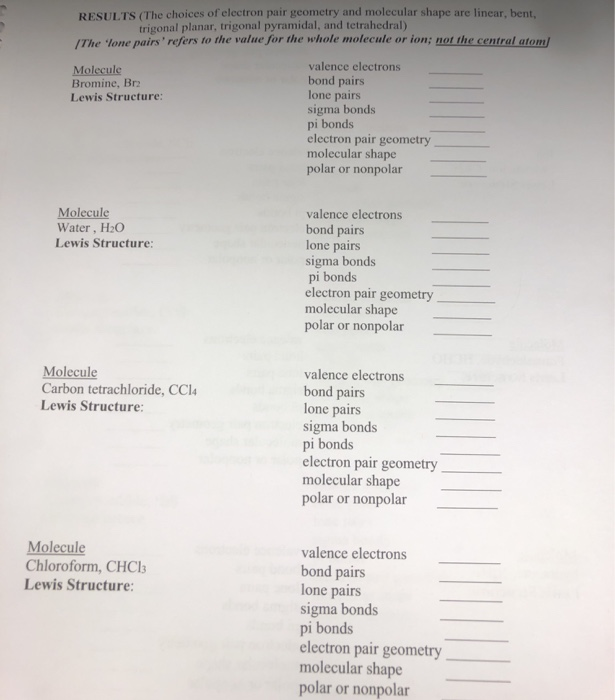RESULTS (The choices of electron pair geometry and molecular shape are linear, bent, trigonal planar, trigonal pyramidal, and tetrahedral) /The lone pairs' refers to the value for the whole molecule or ion; not the central atom/ valence electrons Molecule Bromine, Br bond pairs lone pairs sigma bonds pi bonds electron pair geometry molecular shape polar or nonpolar Lewis Structure: Molecule Water, H2O Lewis Structure: valence electrons bond pairs lone pairs sigma bonds pi bonds electron pair geometry molecular shape polar...

• ### please I need help with this Х Lab 11.pdf Lab 11.pdf INTRODUCTION The chemical and physical...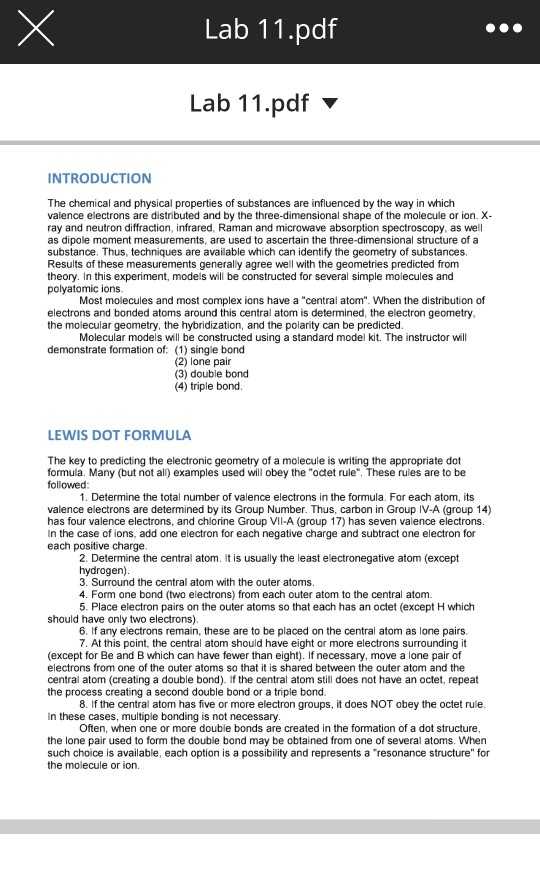please I need help with this Х Lab 11.pdf Lab 11.pdf INTRODUCTION The chemical and physical properties of substances are influenced by the way in which valence electrons are distributed and by the three-dimensional shape of the molecule or ion. X- ray and neutron diffraction, infrared, Raman and microwave absorption spectroscopy, as well as dipole moment measurements, are used to ascertain the three-dimensional structure of a substance. Thus, techniques are available which can identify the geometry of substances Results of...

• ### Provide the correct molecular geometry for (c), given the number of lone pairs and bonding groups...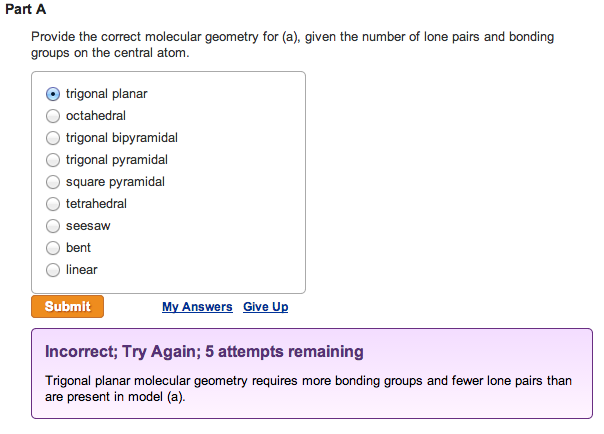Provide the correct molecular geometry for (a), given the number of lone pairs and bonding groups on the central atom. trigonal planar  octahedral  trigonal bipyramidal  trigonal pyramidal  square pyramidal  tetrahedral  seesaw  bent  linearProvide the correct molecular geometry for (b), given the number of lone pairs and bonding groups on the central atom.  trigonal bipyramidal  square pyramidal  bent  tetrahedral  trigonal planar  seesaw  trigonal pyramidal  octahedral  linear Provide the correct molecular geometry for (c), given the number of lone pairs and bonding groups on the...

• ### please I need help with the data sheet INTRODUCTION The chemical and physical properties of substances...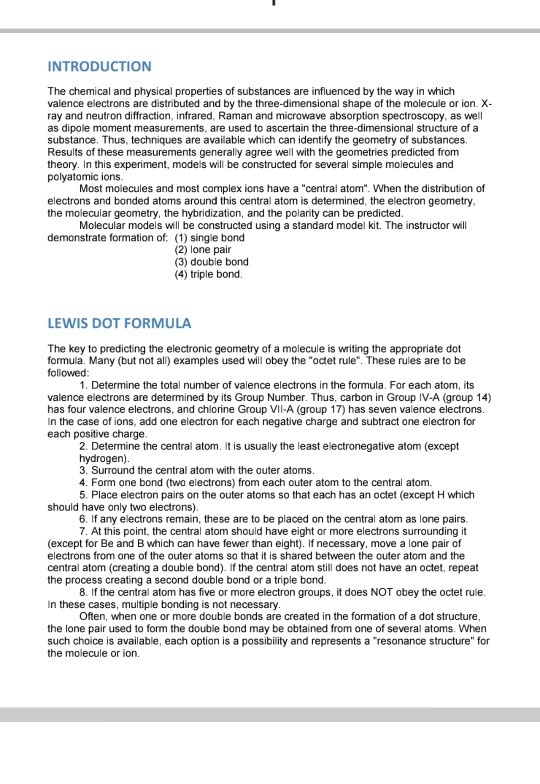please I need help with the data sheet INTRODUCTION The chemical and physical properties of substances are influenced by the way in which valence electrons are distributed and by the three-dimensional shape of the molecule or ion. X- ray and neutron diffraction, infrared, Raman and microwave absorption spectroscopy, as well as dipole moment measurements are used to ascertain the three-dimensional structure of a substance. Thus, techniques are available which can identify the geometry of substances. Results of these measurements generally...

• ### ASF5 1. Lewis Structure 2. Perspective drawing 6. Electronic geometry 3. Steric Number of central 4....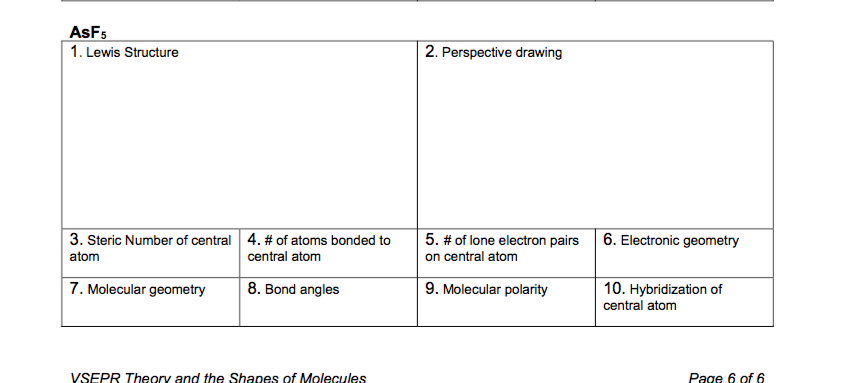ASF5 1. Lewis Structure 2. Perspective drawing 6. Electronic geometry 3. Steric Number of central 4. #of atoms bonded to 5.#of lone electron pairs central atom on central atom atom 7. Molecular geometry 8. Bond angles 9. Molecular polarity 10. Hybridization of central atom Page 6 of 6 VSEPR Theory and the Shapes of Molecules